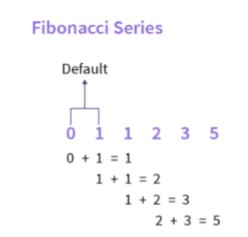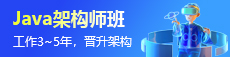# 递归实现斐波那契数列

## 介绍

F(n) = F(n - 1) + F(n - 2)F ( n )=F ( n-1 )+F ( n-2 )其中，F(n)表示n^{第}n时间_斐波那契数。## 示例 1

``````public class FibonacciCalc {
public static int fibRecursion(int count) {
if (count == 0) {
return 0;
}
if (count == 1 || count == 2) {
return 1;
}
return fibRecursion(count - 1) + fibRecursion(count - 2);
}
public static void main(String args[]) {
int fib_len = 9;
System.out.print("Fibonacci Series of " + fib_len + " numbers is: \n");
for (int i = 0; i < fib_len; i++) {
System.out.print(fibRecursion(i) + " ");
}
}
}``````

``````Fibonacci Series of 9 numbers is:
0 1 1 2 3 5 8 13 21``````

## 示例 2

Java 中的斐波那契数与记忆记忆 记忆是一种用于提高递归程序性能的编程技术。在这种技术中，先前计算的结果被存储(缓存)并重复使用。在之前的方法中，我们分别计算每个斐波那契数。但是，在这种方法中，我们将使用之前的结果来计算当前的斐波那契数。因此，如果我们计算了一个数字的斐波那契，我们可以重复使用它而无需再次进行计算。

``````import java.util.HashMap;
import java.util.Map;
public class FibonacciCalc {
static private Map < Integer, Integer > memoizeTable = new HashMap < > ();
// Fibonacci with Memoization
static public int fibMemoize(int n) {
if (n == 0) {
return 0;
}
if (n == 1) {
return 1;
}
if (memoizeTable.containsKey(n)) {
// getting value from the stored result(s)
return memoizeTable.get(n);
}
int result = fibMemoize(n - 1) + fibMemoize(n - 2);
// storing the result in cache
memoizeTable.put(n, result);
return result;
}
public static void main(String[] args) {
//FibonacciCalc fibo = new FibonacciCalc();
System.out.println("Fibonacci value for n = 6 --> " +
fibMemoize(6));
}
}``````

``Fibonacci value for n = 6 --> 8``